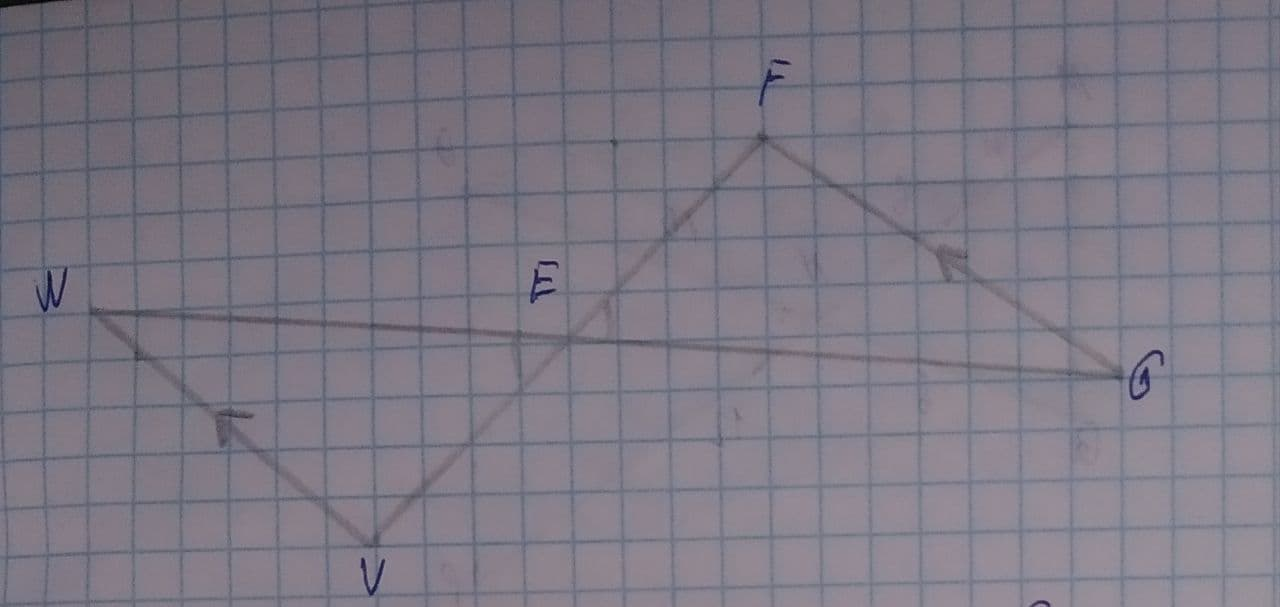State if the triangles are similar. If so state how you know they are similar and complete the similarity statement.rocedwrp 2021-08-04 Answered
State if the triangles are similar. If so state how you know they are similar and complete the similarity statement.• Questions are typically answered in as fast as 30 minutes

Solve your problem for the price of one coffee

• Math expert for every subject
• Pay only if we can solve it2k1enyvp
Step 1
The lines VW and GF are parallel.
And the rest two lines act as traversals
Step 2
$$\displaystyle\angle{G}{E}{F}\stackrel{\sim}{=}\angle{W}{E}{V}$$ (Vertically opposite angles)
$$\displaystyle\angle{E}{F}{G}\stackrel{\sim}{=}\angle{E}{V}{W}$$ (Alternate interior angles because $$\displaystyle{F}{G}{p}{a}{r}{a}{l}\le{l}{W}{V}$$)
$$\displaystyle\angle{F}{G}{E}\stackrel{\sim}{=}{V}{W}{E}$$ (Alternate interior angles)
Hence by AAA criterion
$$\displaystyle\triangle{E}{F}{G}\sim{E}{V}{W}$$